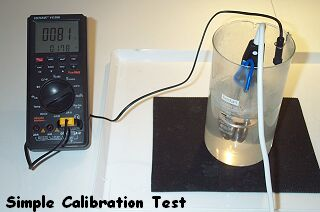Simple test of calibration
created on May 8, 2003 - JLN Labs - Last update May 22, 2003
Toutes les informations et schémas sont publiés gratuitement ( freeware ) et sont destinés à un usage personnel et non commercial
All informations and diagrams are published freely (freeware) and are intended for a private use and a non commercial use.

The purpose of this test is to check the reliability of the method used to measure the Power Input/Output and the efficiency of the Cold Fusion Reactor.

I have used the 1000 mL borosilicate beaker filled with a 600 mL of demineralized water and 16.6 g of Potassium Carbonate ( K2CO3 ), the electrolyte solution used is 0.2 molar ( 0.2 M, ). This is same the electrolyte solution and the same vessel that I have used for the previous tests of the Cold Fusion Reactor v2.0 at High Power.

Test procedure :

1) The temperature of the K2CO3 solution in the vessel has been set initially to 81°C with an electrical heater. ( see below )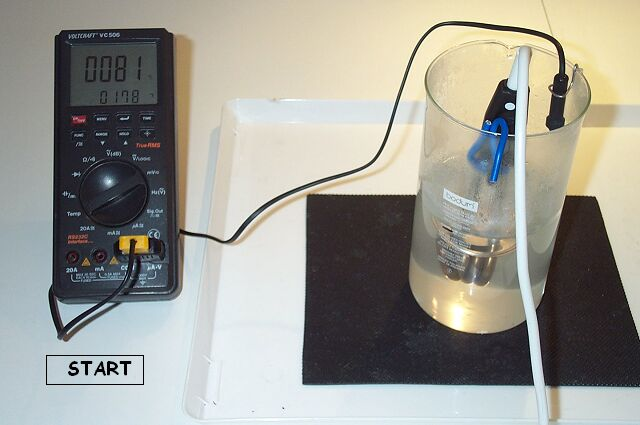2) The weight of the vessel has been measured initially, it was 864 g.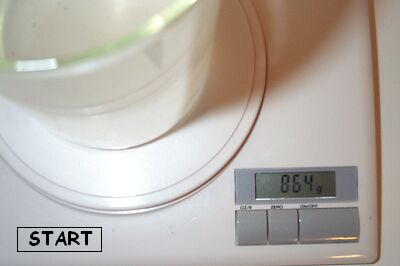3) The electrical power input has been measured with the Power-Meter EKM 265 that I have used in the previous tests ( see cfrtpwr.htm ). The time has been measured with a chronometer.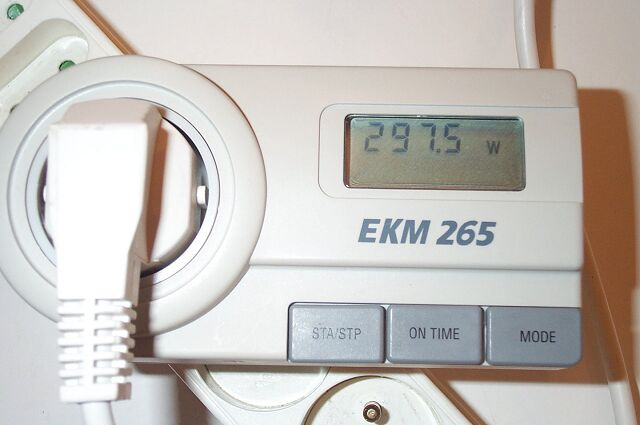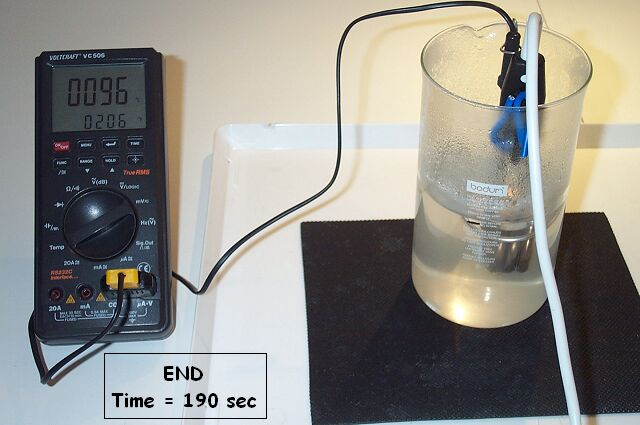4) The final temperature was 96°C after 190 seconds of electrical heating.
5) Then the weight of the vessel has been measured, it was 856 g.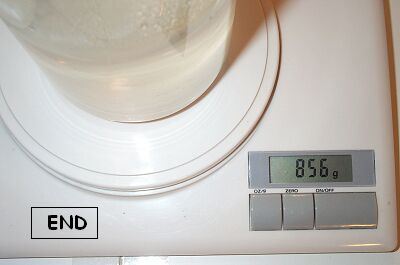The run time of the Electrical Heater has been 190 seconds.

CALIBRATION TEST RESULTS : POWER INPUT/OUPUT and EFFICIENCY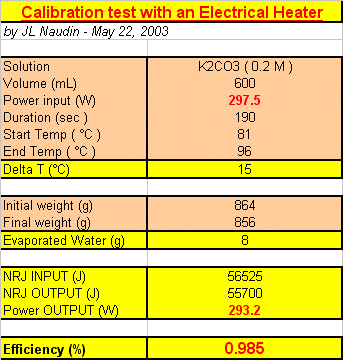The Voltage/Current datas logged give an average electrical power input of 297.5 Watts during 190 seconds, so this gives :

ELECTRICAL ENERGY INPUT = 56525 Joules

The evaporated water in the CFR during the full boiling was 8 mL. We know that we need 2260 J/g to vaporize water. The temperature rise of the 600 mL was 15°C. So, this gives :

ENERGY OUTPUT = ( 8 x 2260 ) + ( 600 x 15 x 4.18 ) = 55700 Joules

 Energy OUTPUT/INPUT = 56525 / 55700 = 0.985

This simple calibration test shows that the method used for the Power Input/Ouput measurements is reliable...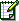Email : JNaudin509@aol.com